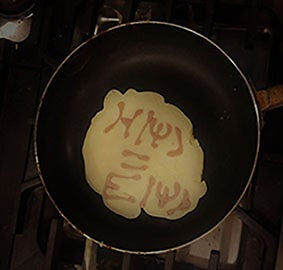# Seminar "An analytical method for solving Schrödinger equation with multivortex initial conditions: applications and extensions to non-linear optics and Bose-Einstein condensates" Dr. Miguel Angel Garcia-March, Valencia Polytechnic University### Date

Tuesday, October 25, 2022 - 14:00 to 15:00

L4E01

### Description

#### Speaker

Dr. Miguel Angel Garcia-March
Distinguished Researcher
Valencia Polytechnic University, Spain

#### Abstract

We present a method to study the dynamics an initial condition which contains many vortices at arbitrary locations propagated with a Schrödinger equation. We consider two systems: i) photonics, where this equation takes the form of a paraxial wave equation, with an initial multisingular vortex Gaussian beam; and ii) Bose-Einstein condensates, where it takes the form of an ideal Gross-Pitaevskii equation. We present first the analytical solution of the dynamics in a homogeneous medium and in a parabolic potential for the ideal non-interacting case (which in photonics is realised with GRIN systems and in BEC by a parabolic trap). We show how to obtain the equations for the trajectories of the positions of phase singularities along with time (the vortex lines). Also, it allows one to predict some quantities of interest, such as the time at which a vortex and an antivortex contained in the initial condition will merge. Secondly, the method is complemented with numerical simulations of the non-linear case. We use a numerical split-step simulation of the non-linear Schrödinger equation to determine how these trajectories and quantities of interest are changed by the presence of interactions. We illustrate the method with several simple cases of interest both in the homogeneous and parabolically trapped or GRIN systems.

Looking forward to seeing many of you at the seminar.
-Quantum Systems Unit / Busch Unit

All-OIST Category: